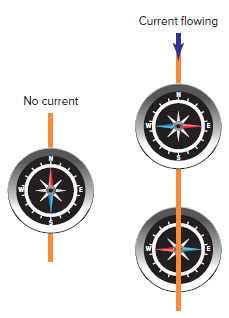Home / Electrical Circuits / Magnetic Field around a Current-Carrying Conductor

Magnetic Field around a Current-Carrying Conductor

Want create site? Find Free WordPress Themes and plugins.

Whenever electrons flow through a conductor, a magnetic field is created around the conductor. This important relationship between electricity and magnetism is known as electromagnetism, or the magnetic effect of current.

When DC current flows, the magnetic field will act in one direction, as illustrated in Figure 1. AC current flow will produce a magnetic field that varies in direction with the direction of the electron current flow.Figure 1 Magnetic field around a current-carrying conductor.

The amount of current flowing through a single conductor determines the strength of the magnetic field it produces. Increasing the current flow increases the strength of the magnetic field. Normally the strength of the magnetic field around a single current-carrying conductor is comparatively weak and therefore goes undetected.

A compass can be used to reveal both the presence and direction of this magnetic field, as illustrated in Figure 2. When the compass is brought close to a conductor carrying DC current, the north-seeking pole of the compass needle will point in the direction the magnetic lines of force are traveling. As the compass is rotated around the conductor, a definite circular pattern will be indicated.Figure 2 Using a compass to trace the magnetic field.

A relationship exists between the direction of current flow through a conductor and the direction of the magnetic field. The left-hand rule for conductors, illustrated in Figure 3, demonstrates this relationship.

If a current-carrying conductor is grasped with the left hand with the thumb pointing in the direction of electron flow (− to +), the fingers point in the direction of the magnetic lines of force or flux.Figure 3 Left-hand conductor rule.

An end view of the wire is sometimes used to simplify the drawing of a conductor that is carrying current, as illustrated in Figure 4. The circle represents the end of the conductor.

Current flow into the conductor is represented by a cross and current flow out by a dot. The direction of the lines of force is shown for two conductors with opposite directions of electron flow. Electron flow away from you (+) creates a counterclockwise magnetic flux pattern. Electron flow towards you () causes a clockwise magnetic flux pattern.Figure 4 End view of a conductor and magnetic field.

The resulting magnetic field produced by current flow in two adjacent conductors tends to cause the attraction or repulsion of the two conductors. If the two parallel conductors are carrying current in opposite directions, the direction of the magnetic field is clockwise around the one conductor and counterclockwise around the other. This sets up a repelling action between the two individual magnetic fields, and the conductors would tend to move apart, as illustrated in Figure 5.Figure 5 Parallel conductors with current flow in opposite directions.

When parallel conductors are carrying current in the same direction, the direction of the magnetic field is the same around each field. The lines of force act in the same direction and link together around both conductors, as illustrated in Figure 6. As a result the two conductors will be attracted to each other and tend to move together. The two conductors under this condition will create a magnetic field equivalent to one conductor carrying twice the current.Figure 6 Parallel conductors with current flow in the same direction.

When a conductor carries a current, it creates a magnetic field which interacts with any other magnetic field present to produce a force. This fact must be taken into consideration when designing large pieces of electrical equipment that handle very high current flows. For example, bus bars carrying high currents must be anchored securely lest they attract each other and short out. Also, short circuit fault currents will increase stress that can result in damage to conductors and raceways if they are not properly secured and protected.

Review Questions

1. What is the relationship between electricity and magnetism?
2. When a compass is used to track a magnetic field, which pole of the compass will point in the direction of the lines of force?
3. What determines the strength of the magnetic field that is produced around a single conductor?
4. Assume two parallel conductors are carrying the same amount of current in the same direction:
1. In what direction will the magnetic forces tend to move the conductors?
2. What is the equivalent strength of the magnetic field created?

Review Questions – Answers

1. Whenever electrons flow through a conductor, a magnetic field is created around the conductor.
2. The north-seeking pole of the compass needle will point in the direction the magnetic lines of force.
3. The amount of current flows through the conductor. The higher the current, the stronger the magnetic field.
4. (a) They would tend to move together. (b) The magnetic field strength would be equivalent to the one conductor carrying twice the current.
Did you find apk for android? You can find new Free Android Games and apps.